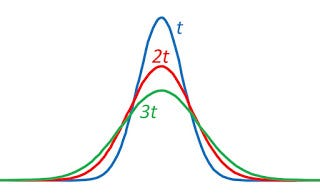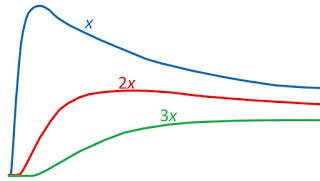# Diffusion From a MicropipetteThe concentration C(x,t) as a function of x at three times t, 2t, and 3t.The concentration C(x,t) as a function of t at three locations x, 2x, and 3x.Random Walks in Biology, by Howard Berg.

Diffusion from a micropipette: A micropipette filled with an aqueous solution of a green fluorescent dye is inserted into a large body of water. At time t = 0, particles of the dye are injected into the water… The total number of particles injected is N… [The diffusion equation] has the solution

This is a three-dimensional Gaussian distribution… Looking through a microscope, one sees the sudden appearance of a green spot that spreads rapidly outward and fades away. The concentration remains highest at the tip of the pipette, but it decreases there as the three-halves power of time.

Section 4.8

Problem 16 ½. When N particles released at time t = 0 and location r = 0 diffuse, the concentration C(r,t) is governed by

(a) Show that this expression for C(r,t) obeys the diffusion equation written in spherical coordinates

(b) Integrate C(r,t) over all space and show that the number of particles is always N.

(c) Calculate the variance (the mean value of r2) and show that σ2= 6Dt, as found in Problem 16. You may need an integral from Appendix K.

(d) Calculate the time tpeak when the concentration at a distance r is maximum.

(e) Calculate the maximum concentration,Cmax, at distance r.

(f) Sketch a plot of C(r,t) as a function of r for three times, and then plot C(r,t) as a function of t for three locations.

--

--